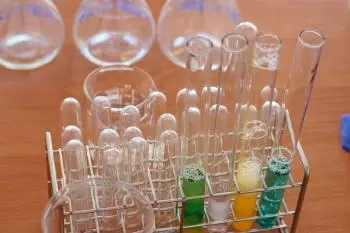# Law of Multiple Proportions: Dalton's LawThe law of multiple proportions is one of the weight laws of chemistry. The law states that if two chemical elements form more than one compound, the proportion of the mass of the second element that combine with a fixed mass of the first element will always be proportions of simple integers.

John Dalton expressed the law of multiple proportions in 1804.

## On What Phenomenon Was Dalton Based That Proust Did Not Realize?

A few years earlier, the French chemist Joseph Proust had proposed the law of definite proportions.

With the works of Prous a phenomenon occurred that Proust did not realize: there are some chemical elements that are related in different proportions to form different chemical compounds. Dalton took advantage of this phenomenon for his research.

The law of definite proportions stated that elements combine to form compounds in certain well-defined proportions with a relationship of whole numbers.

Later, Antoine Lavoisier proved the law of conservation of mass, which helped Dalton.

## What Is the Relationship Between the Law of Multiple Proportions and Dalton's Atomic Model?

Dalton's law was a key proof of atomic theory.

Today, there is a question whether Dalton discovered the law from a hypothesis to investigate the validity of his atomic theory or he discovered it by accident and then explained it using atomic theory.

## What Other Law Is Dalton's Law Confused With?

This law is sometimes called Dalton's law, after John Dalton, the chemist who first expressed it. However, as with Gay-Lussac's law, the name Dalton's law is sometimes used to refer to another law: the law of partial pressures. That is, the calculation of the partial pressures of a gas mixture applying the ideal gas law to each component.

The law of partial pressures states that the total pressure of a mixture of gases is equal to the sum of the pressures that each of them would exert if only one of them occupied the entire volume. This law is fulfilled only if the gases do not react chemically and are at constant temperature.

Author:

Published: December 12, 2021
Last review: December 12, 2021